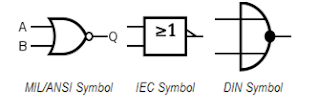## Index

 STA & SI Chapter1 Chapter2 Chapter3 Chapter4 Chapter5 Chapter6 Chapter7 Chapter8 Introduction Static Timing Analysis Clock Advance STA Signal Integrity EDA Tools Timing Models Other Topics

 Extraction & DFM Chapter1 Chapter2 Chapter3 Chapter4 Chapter5 Chapter6 Introduction Parasitic Interconnect Corner (RC Corner) Manufacturing Effects and Their Modeling Dielectric Layer Process Variation Other Topic

## Logic Gates

 Index Chapter1 Chapter2 Chapter3 Chapter4 Digital Background Semiconductor Background CMOS Processing

 1.1 1.2 1.3a 1.3b 1.4 1.5 1.6 Number System Digital Arithmetic Logic Gates Logic Gates Combinational Circuits Multiplex (MUX)

Logic gates are the fundamental building blocks of the digital systems. The name logic gate is derived from the ability of such a device to make decisions. It produces
• One output level when some combinations of input level are present
• A different output level when other combinations of input level are present.

The interconnection of gates to perform a variety of logical operations is called logic design. A logic gate is an electronic circuit with one output and one or more inputs.
Inputs and outputs of  logic gates can occur only in 2 levels. These 2 levels are termed as

 Level 1 Level 2 LOW HIGH FALSE TRUE OFF ON 0 1
There are two types of logic:
1. Positive Level Logic System: Out of the given two voltage levels, the more positive value is assumed as logic ‘1’ and the other as logic ‘0’.
2. Negative Level Logic System : Out of the given two voltage levels, the more negative value is assumed as logic ‘1’ and the other as logic ‘0’.

 Positive Logic Level Negative Logic Level Logic ‘0’ Logic ‘1’ Logic ‘0’ Logic ‘1’ 0V-2V-7V+2V 5V+3V-2V+7V 5V+3V-2V+7V 0V-2V-7V+2V

I am not going to describe each and every logic gate in very detail. For detailing purpose, you can refer the any basics books. Here I am going to summarize most of the basic gates with their Symbol + Truth table + Boolean equation in the form of table.

 Logic Gates Type Distinctive shape Boolean algebra (A & B) Truth table A B Y ANDA.B 0011 0101 0001 ORA+B 0011 0101 0111 NOTA’ 01 10 NAND(A.B)’ 0011 0101 1110 NOR(A+B)’ 0011 0101 1000 XOR/(Exclusive OR)0011 0101 0110 XNOR/(Excluive NOR)0011 0101 1001

Note 1:
• AND, OR and NOT gates are called Basic gates.
• NAND and NOR gates are called Universal gates, because, by using only NAND gates or by using only NOR gates we can realize any gate or any circuit.
• Special gates are Exclusive – OR gate and Exclusive – NOR gate.
• Exclusive – NOR (X – NOR) gate is also called inclusive – OR or Gate of Equivalence.
Note 2 :

• The circuit, which is working as AND gate with positive level logic system, will work as OR gate with negative level logic system.
• The circuit, which is working as OR gate with positive level logic system, will work as AND gate with negative level logic system.
• The number of rows in the truth table is given by 2n where ‘n’ is the number of inputs to the gate.
• The circuit which is behaving as NAND gate with positive level logic system will behave as NOR gate with negative level logic system and vice – versa.
Note 3:
• “The output of AND gate is high if all the input are high”.  (or)  “The output of AND gate is low if any one input is low or all the inputs are low
• “The output of an OR gate is high if any one input is high or all inputs are high”.(or) “The output of an OR gate is zero if all the inputs are zeros”.
• NOT gate is also called inverter.  “The output of a NOT gate is always complement of the input”.
• NAND is nothing but AND gate followed by NOT gate.  “The output of NAND gate is high if any one input or all inputs are low”.
• NOR is nothing but OR followed by NOT gate. “The output of NOR gate is high if all the inputs are low”.  (OR)  “The output of NOR gate is low if any one input is high or all inputs are high”.
• “The output of an X – OR gate is high for odd number of high inputs”.

Above are the basic details of logic gates with few important points to remember. In the next part we will discuss about the realization of basic gates using universal gates (NOR/ NAND) gates. also we will discuss about the Inhibit circuits.

1.the negative logic level table is same as that of positive logic level table??

1.2.sir,
there is a mistake in positive and negative logic table plz correct it if u can,
for others in negative logic just flips the values of logic 0 and logic 1

1.Thanks for pointing ... Corrected.

2.scientist.....

3.4.